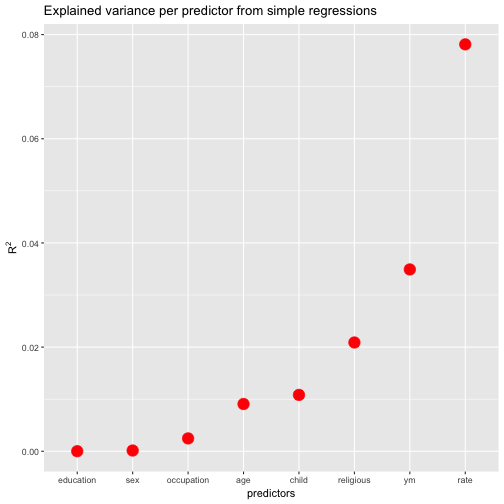# Running multiple simple regressions with purrr

## September 23, 2016

Hadley Wickham’s purrr has given a new look at handling data structures to the typical R user (some reasoning suggests that average users don’t exist, but that’s a different story).

I just tried the following with purrr:

• Meditate about the running a simple regression, FWIW
• Take a dataframe with candidate predictors and an outcome
• Throw one predictor at a time into the regression, where the outcome variable remains the same (i.,e multiple simple regressions (one predictor) where the predictor is changed at each run but the outcome remains the same)
• tidy up the resulting $R^2$ in some nice format.

I found that purrr does the job nicely, and it’s quite instructive, I think. That’s why I wrote it up in this short post:

library(purrr)
library(ggplot2)
library(dplyr)
library(broom)
library(knitr)  # for kable
data(Fair, package = "Ecdat") # extramarital affairs dataset
glimpse(Fair)

## Observations: 601
## Variables: 9
## $sex <fctr> male, female, female, male, male, female, female, ... ##$ age        <dbl> 37, 27, 32, 57, 22, 32, 22, 57, 32, 22, 37, 27, 47,...
## $ym <dbl> 10.00, 4.00, 15.00, 15.00, 0.75, 1.50, 0.75, 15.00,... ##$ child      <fctr> no, no, yes, yes, no, no, no, yes, yes, no, yes, y...
## $religious <int> 3, 4, 1, 5, 2, 2, 2, 2, 4, 4, 2, 4, 5, 2, 4, 1, 2, ... ##$ education  <dbl> 18, 14, 12, 18, 17, 17, 12, 14, 16, 14, 20, 18, 17,...
## $occupation <int> 7, 6, 1, 6, 6, 5, 1, 4, 1, 4, 7, 6, 6, 5, 5, 5, 4, ... ##$ rate       <int> 4, 4, 4, 5, 3, 5, 3, 4, 2, 5, 2, 4, 4, 4, 4, 5, 3, ...
## $nbaffairs <dbl> 0, 0, 0, 0, 0, 0, 0, 0, 0, 0, 0, 0, 0, 0, 0, 0, 0, ...  Fair %>% dplyr::select(-nbaffairs) %>% # exclude outcome, leave only predictors map(~lm(Fair$nbaffairs ~ .x, data = Fair)) %>%
map(summary) %>%
map_dbl("r.squared") %>%
tidy %>%
dplyr::arrange(desc(x)) %>%
rename(r.squared = x) -> r2s

kable(r2s)

names r.squared
rate 0.0781272
ym 0.0349098
religious 0.0208806
child 0.0108181
age 0.0090701
occupation 0.0024613
sex 0.0001377
education 0.0000059

Ok, that appears to be the list of the $R^{2}$ for each simple (one-predictor) regression we have run.

Let’s do a quick sense check with the standard way:

lm1 <- lm(nbaffairs ~ rate, data = Fair)

summary(lm1)

##
## Call:
## lm(formula = nbaffairs ~ rate, data = Fair)
##
## Residuals:
##     Min      1Q  Median      3Q     Max
## -3.9063 -1.3989 -0.5631 -0.5631 11.4369
##
## Coefficients:
##             Estimate Std. Error t value Pr(>|t|)
## (Intercept)   4.7421     0.4790   9.900   <2e-16 ***
## rate         -0.8358     0.1173  -7.125    3e-12 ***
## ---
## Signif. codes:  0 '***' 0.001 '**' 0.01 '*' 0.05 '.' 0.1 ' ' 1
##
## Residual standard error: 3.17 on 599 degrees of freedom
## Multiple R-squared:  0.07813,	Adjusted R-squared:  0.07659
## F-statistic: 50.76 on 1 and 599 DF,  p-value: 3.002e-12

summary(lm1)$r.squared  ##  0.07812718  summary(lm1)$coefficients[2, 4]  #p.value

##  3.002385e-12


Seems to work. To get details of the object summary(lm1), use str(summary(lm1)).

How many did we run? Just the number of columns minus one (the outcome variable).

ncol(Fair)-1

##  8


FWIW, let’s plot the resulting values (and sort the predictors by descending values).

ggplot(r2s, aes(x = reorder(names, r.squared), y = r.squared)) +
geom_point(size = 5, color = "red") +
ylab(expression(R^{2})) +
xlab("predictors") +
ggtitle("Explained variance per predictor from simple regressions")Wait, one more thing. Suppose we are not only interested in $R^{2}$, but in the p-values (OMG). How to get both values from purrr?.

library(magrittr)

Fair %>%
dplyr::select(-nbaffairs) %>%  # exclude outcome, leave only predictors
map(~lm(Fair\$nbaffairs ~ .x, data = Fair)) %>%
map(summary) %>%
map(c("coefficients")) %>%
map_dbl(8)  %>% # 8th element is the p-value
tidy %>%
dplyr::arrange(desc(x)) %>%
rename(p.value = x) -> ps

kable(ps)

names p.value
education 0.9524501
sex 0.7740138
occupation 0.2245709
age 0.0195320
child 0.0107275
religious 0.0003797
ym 0.0000040
rate 0.0000000

Extracting the p-value by map_dbl(8) is surely far from perfect. Any ideas how to better get the value out of this numeric 2*4 matrix? Thoughts are welcome!

### New bar stacking with ggplot 2.2.0

Recently, ggplot2 2.2.0 was released. Among other news, stacking bar plot was improved. Here is a short demonstration.Load libraries`...… Continue reading

#### Crashkurs zur Erstellung von Barplots für Umfrage-Daten

Published on November 13, 2016

#### Some thoughts (and simulation) on overfitting

Published on November 12, 2016